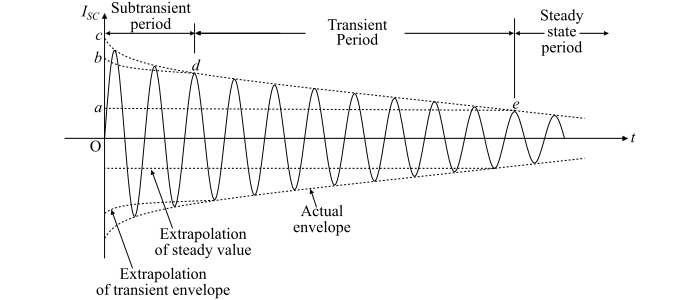# Short Circuit Transient in Synchronous Machine

A sudden 3-phase short-circuit at the armature terminals of a synchronous machine is used to analyse the transient phenomenon. This is the most severe transient condition that can occur in a synchronous generator. It is assumed that the machine is to be initially unloaded and to continue operating at synchronous speed after short-circuit occurs.

The machine being producing normal voltage under no-load condition and their instantaneous values are given by,

$$\mathrm{𝑒_{𝑅} = 𝐸_{𝑚}\:sin\:𝜔𝑡}$$

$$\mathrm{𝑒_{𝑌} = 𝐸_{𝑚}\:sin(𝜔𝑡 − 120°)}$$

$$\mathrm{𝑒_{𝐵} = 𝐸_{𝑚}\:sin(𝜔𝑡 + 120°)}$$

Since the machine is initially unloaded, the only pre-disturbance current in the machine is the field current. When the rotor rotates, each phase sees a resultant time varying flux linkage.

Now, if the armature terminals are short-circuited, a large transient current will flow through them. According to the constant flux linkage theorem, the currents flowing through the armature windings maintain their flux linkage constant at the prefault values. The transient current in each phase consists of an AC component and a DC component. Since, the three phase voltages are displaced by 120°, thus short-circuit occurs at different points on the voltage waves of each phase. As a result, the DC component of the armature current is also different in each phase.The above figure shows the AC component of the symmetrical short circuit current and it may be divided into roughly three periods viz. subtransient period, transient period and steady-state period.

## Subtransient Period

The subtransient period lasts for only about 2 cycles after the fault occurs. During this period, the current in the armature is very large and decays very rapidly. The RMS value of initial current, which is the current at the instant of short-circuit, is known as the sub-transient current and is denoted by I”.

The time constant of the sub-transient current is denoted by τ” and it is determined from the slope of the sub-transient current. The reactance of the winding corresponding to I” is called the sub-transient reactance (${𝑋^{"}_{𝑑}}$). The sub-transient reactance is due to the presence of damper windings. If $E_{0}$ is the open-circuit phase voltage, then

$$\mathrm{Subtransient\:reactance,\:{𝑋^{"}_{𝑑}} =\frac{𝐸_{0}}{𝐼^{"}}}$$

Where I” is the subtransient current without DC offset. This current is about 5 to 10 times of the rated current. Hence, during the subtransient period, there is a large initial current flows in the armature. This current lags 90° behind the voltage.

The subtransient current produces a large demagnetising MMF in the direct axis, which tends to reduce the main field pole MMF. But, the main field flux cannot decrease suddenly because the stored energy associated with this flux takes some time to dissipate.

Also, according to the constant-flux linkage theorem, currents are induced in the field and damper windings which will try to maintain the flux linkage conditions in the machine exactly as they were at the instant of short-circuit at the armature terminals. Since, these transients disappear after few cycles, thus these are called subtransients.

## Transient Period

The transient period is the period of time during which current falls at a slow rate. The transient period lasts for about 30 cycles. During this period, current flowing in the machine is called the transient current and is denoted by $I^{'}$. The transient current is caused by a DC component of current induced in the field winding at the instant of short-circuit. The transient period is much greater than the subtransient period because the time constant of the DC field winding is much greater than the time constant of the damper windings. The time constant of the transient current is denoted by $τ^{'}$.

The value of the RMS current during the transient period is about 5 times of the steady-state fault current. The reactance of the winding corresponding to I’ is called the transient reactance and is denoted by ${𝑋^{'}_{𝑑}}$. Now, if E0 is open-circuit phase voltage, then

$$\mathrm{Transient\:reactance,{𝑋^{'}_{𝑑}}=\frac{𝐸_{0}}{𝐼^{′}}}$$

After the transient period, the fault current reaches its new steady-state value. The steady state current is denoted by $I_{ss}$. The reactance corresponding to $I_{ss}$ is called synchronous reactance ($X_{d}$) and is given by,
$$\mathrm{Synchronous\:reactance,\:𝑋_{𝑑} =\frac{𝐸_{0}}{𝐼_{𝑠𝑠}}}$$
Where, $E_{0}$ is the open circuit phase voltage.
$$\mathrm{𝐼_{𝑠𝑐}(𝑡) = (𝐼^{"} − 𝐼^{′})𝑒^{−𝑡⁄𝜏"} + (𝐼^{′} − 𝐼_{𝑠𝑠})𝑒^{−𝑡⁄𝜏′} + 𝐼_{𝑠𝑠}}$$